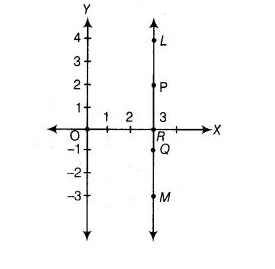# In figure LM is a line parallel to the Y-axis at a distance of 3 units.`
Question:

In figure LM is a line parallel to the Y-axis at a distance of 3 units.

(i) What are the coordinates of the points P, R and Q?

(ii) What is the difference between the abscissa of the points L and M?Solution:

Given, LM is a line parallel to the Y-axis and its perpendicular distance from Y-axis is 3 units.

(i) Coordinate of point P = (3, 2) [since, its perpendicular distance from X-axis is 2] Coordinate of point 0 = (3, -1) [since, its perpendicular distance from X-axis is 1 in negative direction of Y-axis]. Coordinate of point R = (3, 0) [since its lies on X-axis, so its y-coordinate is zero].

(ii) Abscissa of point L = 3, abscissa of point M = 3
Difference between the abscissa of the points L and M = 3-3 = 0